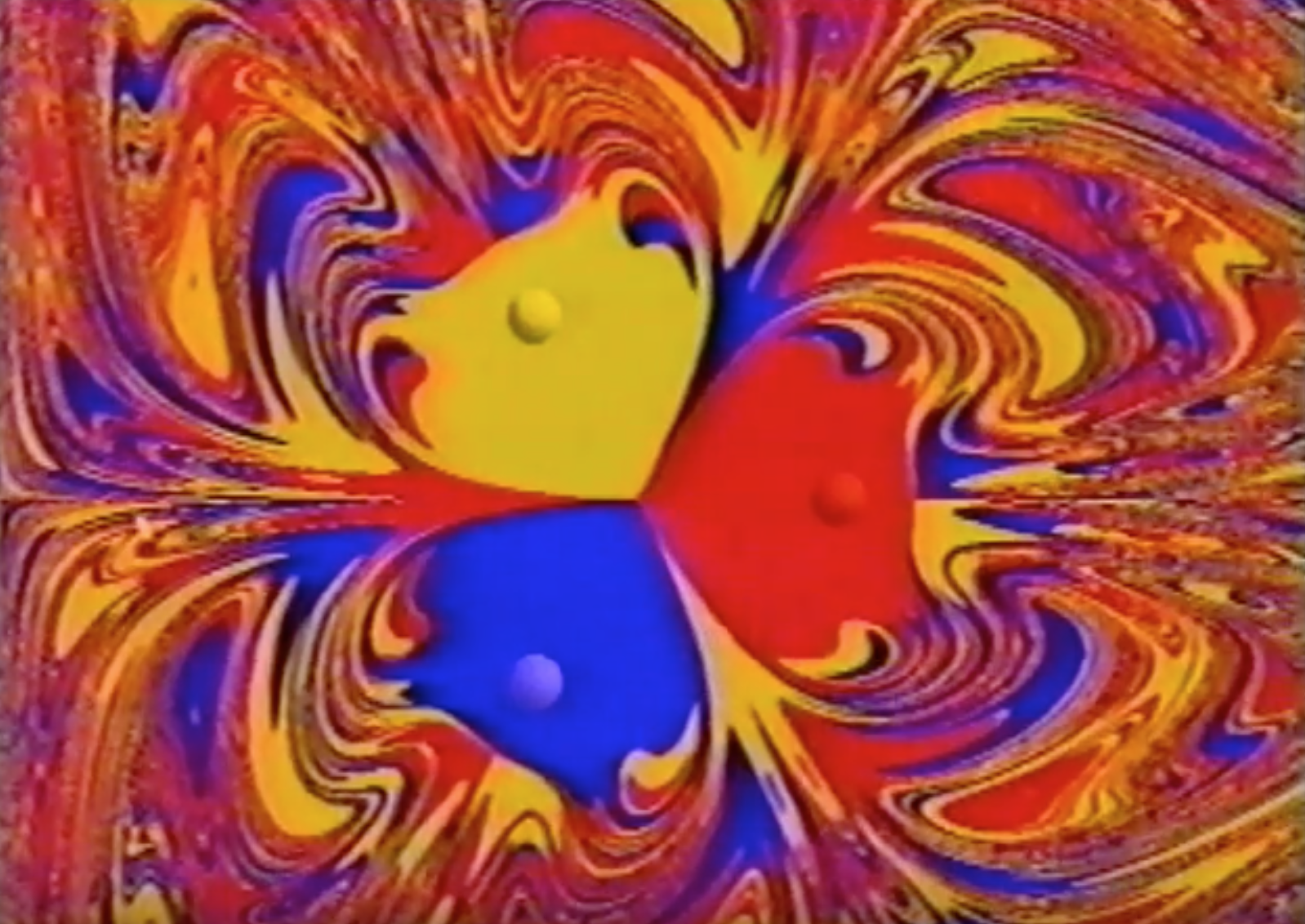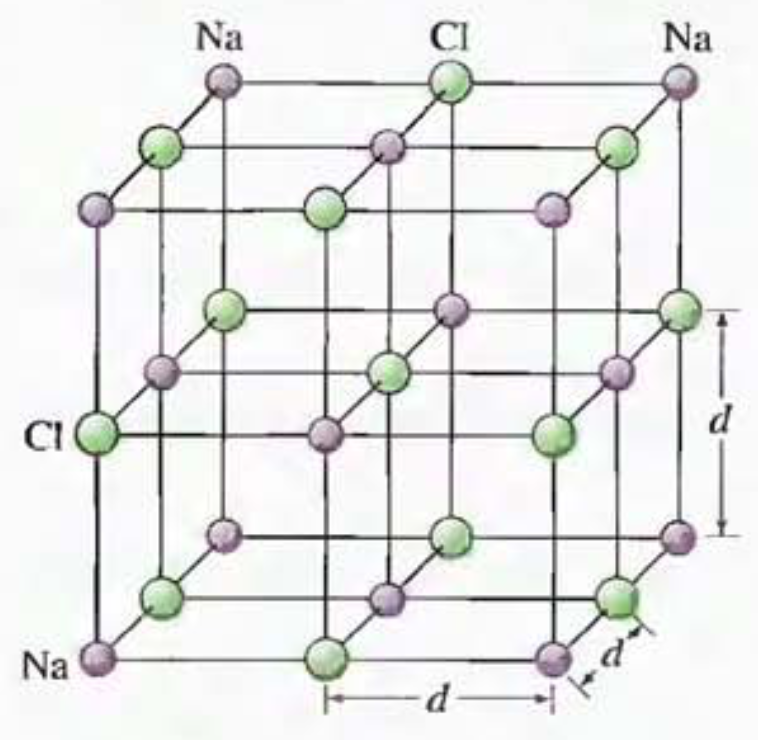#### 2018/2019 board members of the Istanbul Toastmasters (ITM) Club.### Oscillations, Orders

In the universe, everything is in motion. There are repetitions in this motion. These repetitions are sometimes exactly periodic, which I will call as harmonic motion, sometimes in more complex order.

Imagine a ball attached to a spring, and the spring is hanged on a plate. When you pull down the ball and release, it starts to oscillate up and down. Roughly, the more force you apply, the more energy it gains. Independently of force that you apply to the ball, namely at all energy values, its motion will be harmonic oscillatory motion. Now, it is oscillating. As time goes by, it gradually damps and finally stops. Its minimum energy is zero.

We can give other examples to this motion. For example, swinging pendulum or an object turning around another object.

Let's take a harmonic oscillatory particle and minimize its size till very small scale. Now, we have an extremely small one. In this scale, nothing can stay motionless. Even the minimum energy of a particle is not zero. I will call minimum energy level as zero-point. Its name is zero-point but its energy is not zero. Because in this case, the particle fluctuates between in existence and extinction, again in harmonic oscillatory motion. Same characterized motion continues in a different context.

Except harmonically oscillating particle, more complex many-body systems also fluctuate at zero-point with random fluctuations or coherent fluctuations.

Also in vacuum, namely in nothingness, there are fluctuations. Energy is borrowed from nothing, particle is created, later energy has to be repaid and the particle is annihilated. These take place in very short time interval.

In absolute zero temperature, namely the point where motion ends, everything are expected to be at rest and aliveness finishes, there are fluctuations. This means that physical things and life can still occur.

Everything's source is vibrations. Building blocks of matter vibrates. As an elementary particle an electron is the same as all other electrons. So how do we get distinguishable substances? From limited number of elementary particles, the thing that creates distinguishable individuals or objects is their quantum states. And one of the contributions to create distinguishing quantum states comes from the particle's vibrations.

As not literally vibration but from the viewpoint of repetition or order, nature's behaviour is like this. For example, take an iron ball attached to a rope. Put 3 magnets under this pendulum. Magnets are located at the corners of an imaginary equilateral triangle. Our pendulum is above and at the center of the triangle at rest. When we pull the ball and release, it turns around and stops over one of the magnets. When we pull and release the ball from different locations, after swinging around it stops over one of them. When we analyze the starting and finalizing points of the ball, we see that the pattern/picture is fractal. Within the framework of the experiment, completely chaotically seeming behaviour in fact satisfies a fractal structure.Fractal is a repeating structure as going into smaller scale. We can see this structure in the branching of trees, rivers, our lungs, blood vessels etc.An example from chemistry is a mixture of certain solutions which has reactions (Belousov-Zhabotinsky) shortly called as BZ reactions. In time, on the surface of this solution, circular and spiral waves take place (figure \ref{fig:BZandCow}). These movements seem to happen randomly but are governed by certain complex dynamics. Another example is black blotches occurred on the skin of cows. In nature, there are orders and repetitions at different levels.As a conclusion, in the zero-point of a pendulum there is still harmonic oscillatory motion. In the zero-point of complex systems there are fluctuations. In vacuum and absolute zero temperature there are fluctuations. Seemingly chaotic nature namely its behaviour, in fact satisfies a kind of regularity or an order. All these help us to understand and predict the behaviour of nature.

### Particle-Wave Duality: Matter Waves

The scale that comprises the basic unit of objects is the quantum scale. In this scale, everything has particle-wave duality. Examples of wave behavior exhibition are sound waves, water waves and electromagnetic waves. However, everything has mass and occupies space is matter and exhibits particle behavior. Particle-wave duality is the observing of both properties on same object. This duality firstly discovered on light. Light exhibits particle behavior when you setup measurement testing its particle properties. It behaves as wave when you measure its wave properties. Which property will be demonstrated by the object depends on your experimental setup. Namely, you (the observer) decide on the entity's role.

Photon (the quanta or basic unit of light) is a massless entity. However, the term matter waves is asserted by physicist Louis de Broglie and is used for all massive objects, from atomic to macroscopic. The condition to observe the wave characteristic of a matter is the detection of wave properties which are interference, reflection, diffraction and scattering.

We mentioned about 3 different waves. Electromagnetic and mechanical waves (like water and sound waves) is the classification made on the basis of ability or inability to transfer energy in vacuum. They are classified according to the medium that they require to transfer energy. However de Broglie waves are probability waves and they are completely quantum mechanical, but their effects are physical. They are the waves before the object/entity is materialized. de Broglie wave represents us a probability distribution about the position of an object. It is related to the materialization and localization position of an object. It determines at what probability on which position, entity will come out.

There is a universal relationship between the wavelength and momentum of an object. Momentum is mass times speed:

$$p=m v$$

Wavelength is denoted by $$\lambda$$. Their relation is identified by the equation

$$\lambda=\frac{h}{p}$$

$$h$$, naming as Planck's constant, contains energy unit. It is the minimum measurable or observable amount of energy in this universe. If there are smaller amounts, they will have no meaning physically. Now we will continue to matter waves and later back to this constant.

Assume you are running with a speed of 10 m/s. Your weight is 70 $$kg\frac{m}{s^2}$$ so your mass is approximately 7 kg. When we substitute your mass and speed into the equation, we obtain a result on the order of $$10^{-36}$$ m. Your wavelength is much smaller than any aperture through which you can pass. Your wave can pass any aperture as a whole without diffraction. That's why, we cannot observe the wave properties of large-scale objects. To observe wave behavior, we must observe wave properties like diffraction, interference or reflection.

On the other hand, if we take an electron moving at $$10^{6}$$ m/s and with mass $${\sim}10^{-31}$$ kg, we see that its wavelength is on the order of $$10^{-10}$$ m which can be observed on crystalline structures. Generally, crystalline structures have regular spacing of atoms and the spacing distance is on the order of electrons' wavelength. Working with electrons and crystalline structure result in observing electrons' waves.

For example, table salt NaCl, has atoms in regular arrays and atomic spacing on the order of $$10^{-10}$$ m. When we bombard NaCl with electrons, we observe diffraction and interference pattern.Distances must be comparable to observe wave behavior effects.

Planck's constant affects the observability of wavelike behavior. For a given mass and speed, it determines the wavelength in direct proportion.

$$\lambda=\frac{h}{p}$$

$$\uparrow=\frac{\uparrow}{\longleftrightarrow} \hspace{1cm} \downarrow=\frac{\downarrow}{\longleftrightarrow}$$

If we could scale the constant $$h$$ according to ourselves, we can carry the wave properties to macro scale or if we could be in very small masses, we would also observe wavelike behavior on ourselves in quantum scale. Now we have a concrete shape but in the other situation we would have different depiction. This would lead to some phenomena like uncertainties. For example if we could behave wavelike, our position in space and momentum could not be detectable with same accuracy at the same time or we could violate energy conservation for a short time. I think this depiction of world would be interesting.